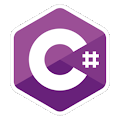Programming Course in C# ¡Free!

# Multiples

Proposed exercise

Create a program to write on screen the numbers from 1 to 500 that are multiples of 3 and also multiples of 5 (hint: use the remainder of the division).

Output

Solution

```using System;
public class Multiples
{
public static void Main()
{
int i = 1;

for (i=1; i <= 500; i++)
{
if ((i%3 == 0) && (i%5 == 0))
Console.Write("{0} ",i);
}
}
}
```